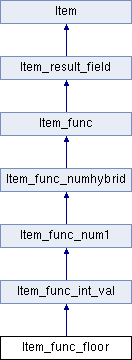My Project
Item_func_floor Class Reference
Inheritance diagram for Item_func_floor:List of all members.

## Public Member Functions

Item_func_floor (Item *a)
const char * func_name () const
longlong int_op ()
Performs the operation that this functions implements when the result type is INT.
double real_op ()
Performs the operation that this functions implements when the result type is REAL.
my_decimaldecimal_op (my_decimal *)
Performs the operation that this functions implements when the result type is DECIMAL.
bool check_partition_func_processor (uchar *int_arg)

## Member Function Documentation

 my_decimal * Item_func_floor::decimal_op ( my_decimal * ) ` [virtual]`

Performs the operation that this functions implements when the result type is DECIMAL.

Parameters:
 A pointer where the DECIMAL value will be allocated.
Returns:
• 0 If the result is NULL
• The same pointer it was given, with the area initialized to the result of the operation.

Implements Item_func_numhybrid.

 longlong Item_func_floor::int_op ( ) ` [virtual]`

Performs the operation that this functions implements when the result type is INT.

Returns:
The result of the operation.

Implements Item_func_numhybrid.

 double Item_func_floor::real_op ( ) ` [virtual]`

Performs the operation that this functions implements when the result type is REAL.

Returns:
The result of the operation.

Implements Item_func_numhybrid.

The documentation for this class was generated from the following files: